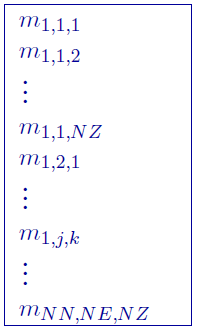3.3.4. Model file¶

This file contains the cell property values (SI) of the model and is the most common of the model files. Inversion models (forward, initial, reference, recovered, and lower and upper bounds) are in this format. The following is the file structure of the model fileEach $$m_{i,j,k}$$ is the property in the $$[i,j,k]^{th}$$ model cell. $$[i, j, k]=[1, 1, 1]$$ is defined as the cell at the top, south-west corner of the model. The total number of lines in this file should equal $$NN \times NE \times NZ$$, where $$NN$$ is the number of cells in the north direction, $$NE$$ is the number of cells in the east direction, and $$NZ$$ is the number of cells in the vertical direction. The model ordering is performed first in the z-direction (top-to-bottom), then in the easting, and finally in the northing.

NOTE: Only the cells completely below the (interpolated) topographic surface are kept within an inversion. The cells above or at the topographic surface are removed from the model, although these must still be included in the as if they are a part of the model. For input model files these cells can be assigned any value. The recovered model produced by inversion program also includes the cells that are excluded from the model, but these cells will have unrealistic values set to -100.

3.3.4.1. Active cells file¶

This file is optional. The active cells file contains information about the cells that will be incorporated into the inversion. It has exact same format as the , and thus must be the same size, with one exception. Values of this file are restricted to either -1, 0 or 1. By default, all cells below the earth’s surface are active (1) and incorporated into the inversion. Inactive cells are set to the values of the reference model and influence the forward modelling. There are two kinds of inactive cells:

• inactive cells that do not influence the model objective (set to 0) and
• inactive cells that do influence the model objective function (set to -1).